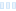Does anyone know how to write the colouring algorithm in the following link: https://fractalforums.org/index.php?topic=368.msg27841#msg27841
into Ultra Fractal code? Some help would be appreciated.

Does anyone know how to write the colouring algorithm in the following link: https://fractalforums.org/index.php?topic=368.msg27841#msg27841 into Ultra Fractal code? Some help would be appreciated.

0

The difficulty is that the coloring formulas only have access to the series of z values generated by the fractal formula. Thus the fractal formula has to keep track of the true z within itself, do some extra calculations, and store the needed result in z for the coloring formula to use in its summation. Something similar is done with various Slope fractal formulas, as in dmj.ufm and reb.ufm.

Thus one would have to write a cooperating pair, fractal formula and coloring formula.

The difficulty is that the coloring formulas only have access to the series of z values generated by the fractal formula. Thus the fractal formula has to keep track of the true z within itself, do some extra calculations, and store the needed result in z for the coloring formula to use in its summation. Something similar is done with various Slope fractal formulas, as in dmj.ufm and reb.ufm. Thus one would have to write a cooperating pair, fractal formula and coloring formula.

0
28
views
1
replies
2
followers
live preview
Enter at least 10 characters.
WARNING: You mentioned %MENTIONS%, but they cannot see this message and will not be notifiedSaving...
Saved
All posts under this topic will be deleted ?
Pending draft ... Click to resume editing